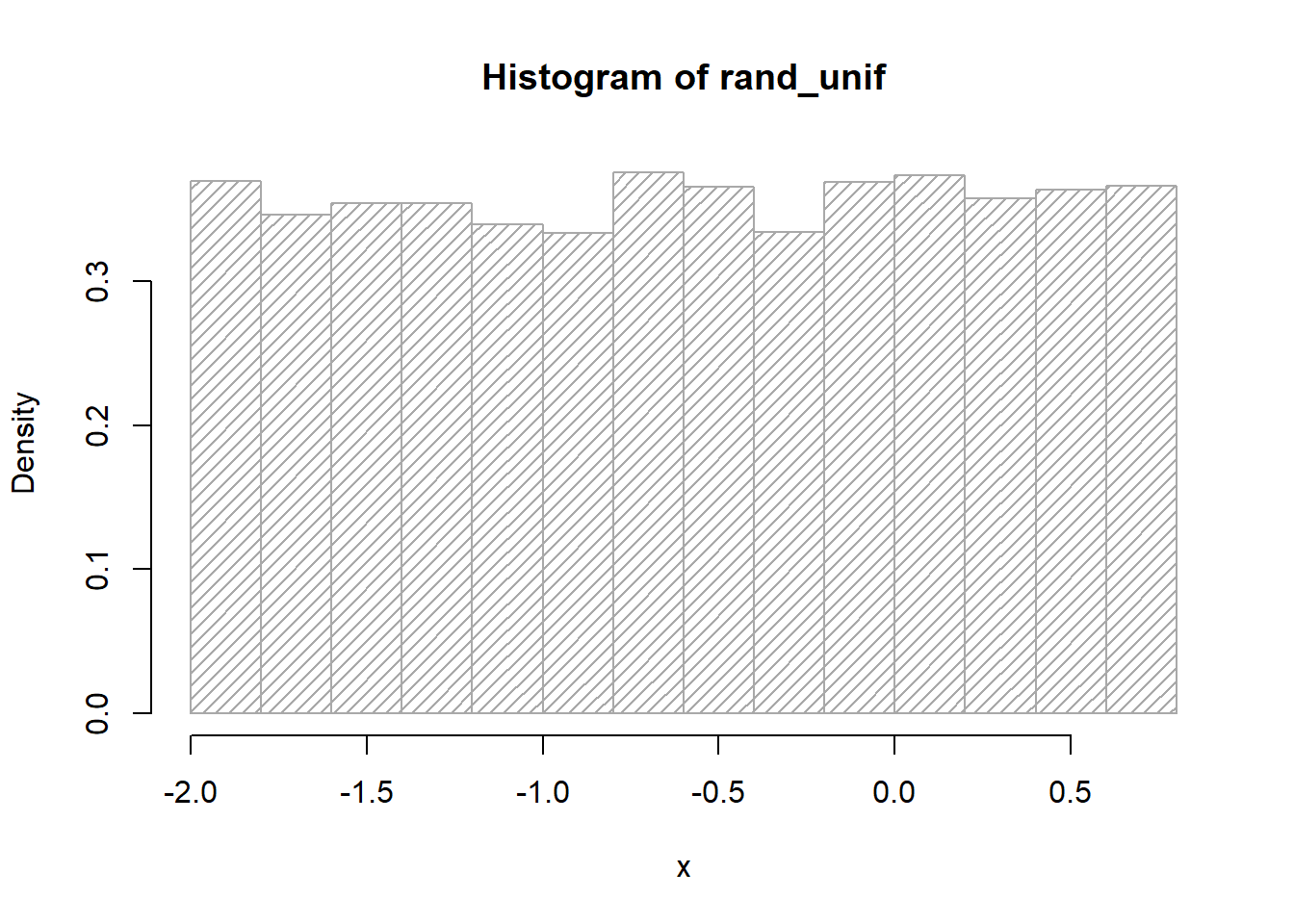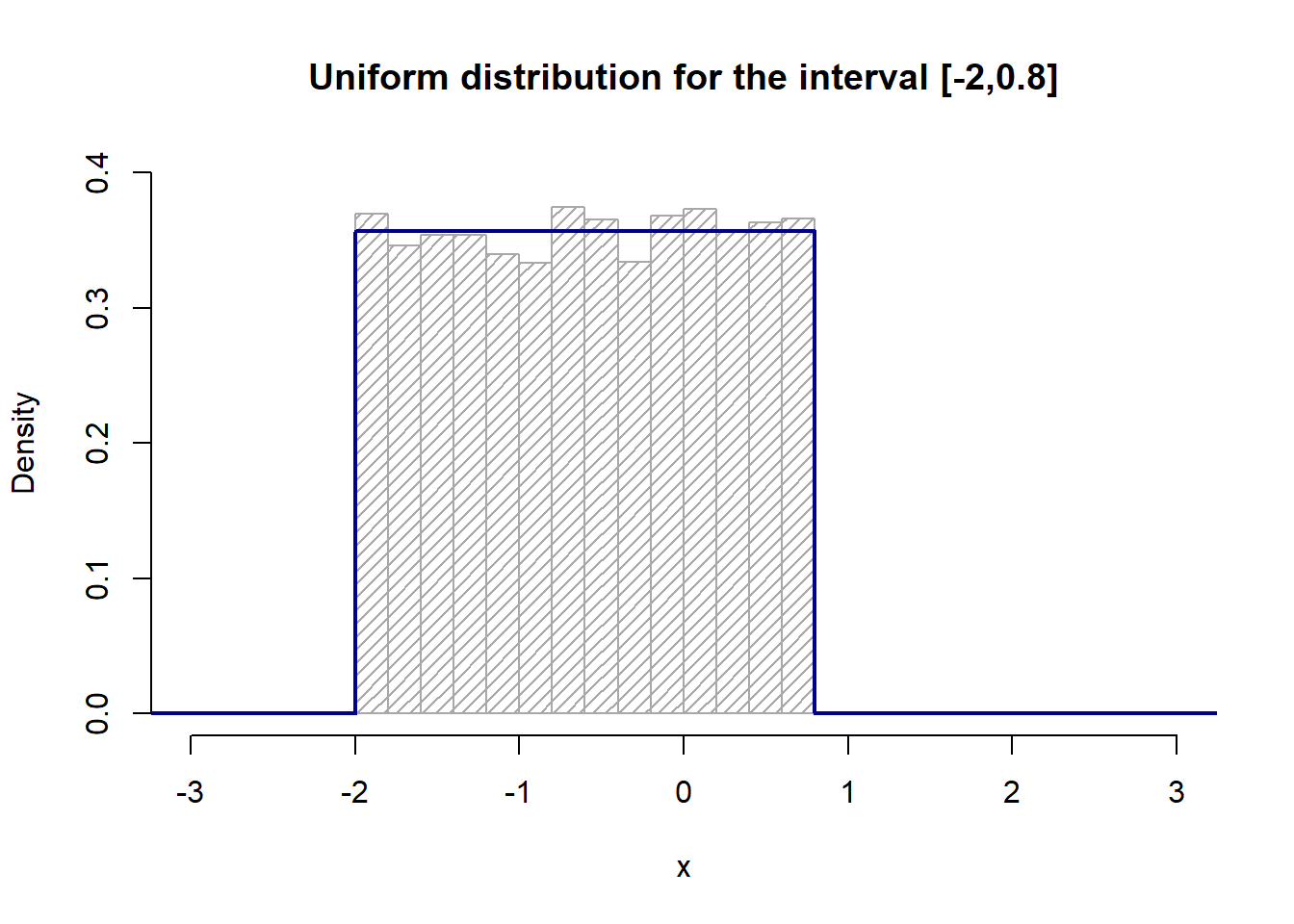The R software provides access to the uniform distribution by the dunif(), punif(), qunif() and runif() functions. Apply the help() function on these functions for further information.

The runif() function generates random deviates of the uniform distribution and is written as runif(n, min = 0, max = 1). We may easily generate a number of n random samples within any interval, defined by the min and the max argument.

# generate 40 random variables, uniformly distributed between -1 and 1
runif(40, min = -1, max = 1)
##    0.19385536 -0.42233526 -0.75538268  0.22654428 -0.11468464  0.98650747
##   -0.27771449  0.74859086  0.47980420 -0.60683451 -0.79377336  0.06917257
##  -0.65462312 -0.02456246 -0.17790175  0.31869804 -0.61292911 -0.32424434
##  -0.36463871  0.98544770 -0.97368184  0.04194384  0.39385372  0.32302810
##  -0.77328663  0.86339500 -0.31844080  0.16948710 -0.09653187 -0.16704798
##   0.78025444  0.73748447  0.12908346  0.40267567 -0.38549169 -0.72528639
##  -0.73963738 -0.94049493  0.72430238  0.81898918

We may approximate the density function for $$X \sim U(-2, 0.8)$$ by using the runif() function and plot the results as a histogram:

rand_unif <- runif(10000, min = -2, max = 0.8)
hist(rand_unif, freq = FALSE, xlab = "x", density = 20, col = "darkgray")Further, we plot both, the density histogram from above, as well as the uniform probability distribution for the interval [-2,0.8], by applying the dunif() function:

a <- -2
b <- 0.8
hist(rand_unif,
freq = FALSE,
xlab = "x",
ylim = c(0, 0.4),
xlim = c(-3, 3),
density = 20,
main = "Uniform distribution for the interval [-2,0.8]",
col = "darkgray"
)
curve(dunif(x, min = a, max = b),
from = -5, to = 5,
n = 100000,
col = "darkblue",
lwd = 2,
yaxt = "n",
ylab = "probability"
)The figure indicates that the 10,000 samples randomly drawn from a uniform distribution (histogram plot) approximate the uniform probability distribution $$X \sim U(-2, 0.8)$$ (line plot) well.

Further, we can use the punif() function to calculate the area under the curve for a given threshold value. Or, we can use the qunif() function to return a threshold value for a particular probability.

#### Exercises

Consider the uniform probability distribution given by $$X \sim U(-3, 5.5)$$.

Question 1: What is the mean, $$\mu$$, for the given uniform distribution?

### your code here
Show code
unif_mean <- (-3 + 5.5) / 2
unif_mean
##  1.25

The mean, $$\mu$$, for the uniform probability distribution given by $$X \sim U(-3, 5.5)$$ is 1.25.

Question 2: What value of $$x$$ divides the given uniform distribution into two equal parts? Or, written more formally $$P(X<?) = 0.5$$.

### your code here
Show code
px_50 <- qunif(0.5, min = -3, max = 5.5)
px_50
##  1.25

Not a surprise at all. The value of $$x$$ that divides the uniform distribution into two equal parts is 1.25 and is thus equal to $$\mu$$.

Question 3: Given that the distribution from above describes a physical phenomenon. If we take a measurement of that physical process governing the phenomenon, what is the probability of measuring a value $$>=4$$, or written more formally $$P(X>=4)$$? Owing to the nature of a uniform distribution the measurement of any particular value within the interval $$[-3, 5.5]$$ is equally likely.

We will solve that question in two ways, numerically and analytically. First, in order to solve the question numerically we need to conduct an experiment. We will repeat our measurement for a large number of times and then count the number of times we registered a value $$>= 4$$. Thanks to the power of R and the integrated random number generator (runif() for uniformly distributed data) the repetition task is very easy. However, be aware that in real life applications quite often there is only a very limited number of measurements available.

measurements <- runif(10000, min = -3, max = 5.5) # take 10,000 measurements
above_threshold <- sum(measurements >= 4) # count the number of values >= 4
above_threshold / length(measurements) # calculate the proportion of values >= 4
##  0.173

The results shows that approximately 17% of measurements yield values $$>=4$$.

Second, in order to solve the question analytically, we make use of the cumulative probability density function, which is implemented in R for uniform distributions by the punif() function. Make sure to set the lower.tail argument to lower.tail = FALSE, as we are looking for the probability to measure values $$>= 4$$, thus we are interested in the area under the curve right to the value of $$x = 4$$.

### your code here
Show code
result <- punif(4, min = -3, max = 5.5, lower.tail = FALSE)
result
##  0.1764706

The analytic approach yields a result of 0.1764706 or, in other words, with a probability of 17.65 % we obtain values $$>= 4$$. Thus, $$P(X>=4)\approx 0.18$$.

Obviously, both approaches yield very similar results. However, be aware that the result of the numerical approach is an approximation to the analytic result. Keep in mind that the quality of such an approximation is very sensitive to the number of random variables constituting the sample, in our case the number of measurements.